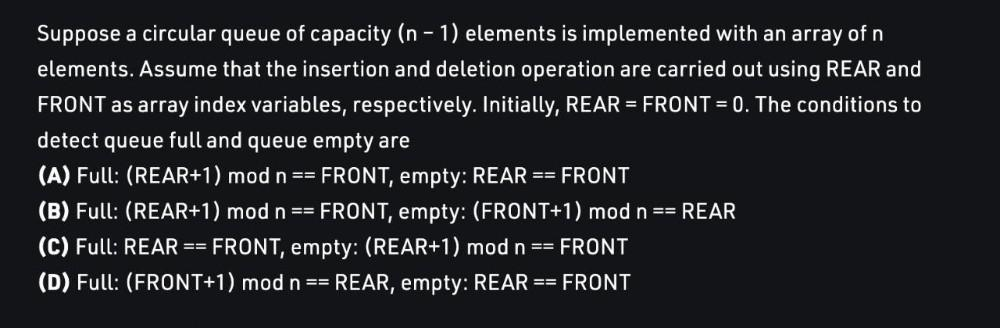Question:

# Suppose a circular queue of capacity (n-1) elements is implemented with an array of n elements. Assume that the insertion and deSuppose a circular queue of capacity (n-1) elements is implemented with an array of n elements. Assume that the insertion and deletion operation are carried out using REAR and FRONT as array index variables, respectively. Initially, REAR = FRONT = 0. The conditions to detect queue full and queue empty are (A) Full: (REAR+1) mod n == FRONT, empty: REAR FRONT (B) Full: (REAR+1) mod n FRONT, empty: (FRONT+1) mod n == REAR (C) Full: REAR == FRONT, empty: (REAR+1) mod n == = FRONT (D) Full: (FRONT+1) mod n == REAR, empty: REAR FRONT == == ==